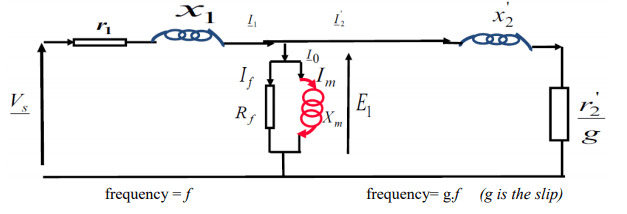# Course_question

EXERCISE: COURSE QUESTION

Given the simplified scheme of the induction machine per phase and neglecting ( r1 + jx1)×I1, prove that the magnetizing current IM=Vs/f×(1/2π×LM). If we want to work at low frequency, are our simplifying assumptions acceptable? If not justify.CORRECTIONThe figure above represents the equivalent phase diagram of an induction machine. The current flowing in the magnetising inductor creates the flux : LM = XM
Optimum engine performance is achieved if the flux, and thus the magnetizing current, is kept substantially constant over the entire speed range. The magnetizing current can be calculated by the expression: IM = E1/LM .

with  E1 = Vs – ( r1 + jx1)×I1 ; Neglecting  ( r1 + jx1)×I1;   We obtain: Im = Vs/f (1/2π×LM)

The magnetizing current can therefore be kept constant while keeping the ratio substantially constant. However, at low frequencies and voltages, the term ( r1 + jx1)×I1 can no longer be neglected, and in particular the term r1I1.